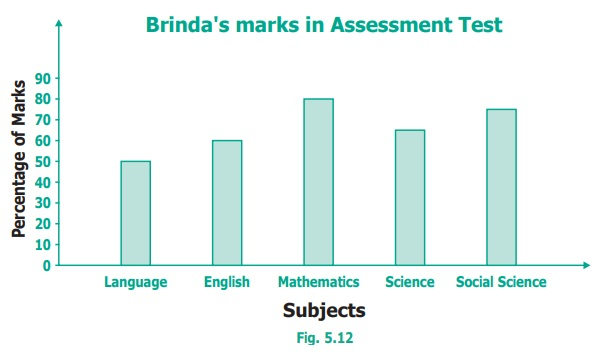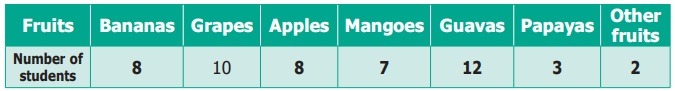Home | | Maths 6th Std | Exercise 5.3

# Exercise 5.3

6th Maths : Term 1 Unit 5 : Statistics : Exercise 5.3 : Text Book Back Exercises Questions with Answers, Solution

Exercise 5.3

1. Read the given Bar Graph which shows the percentage of marks obtained by Brinda in different subjects in an assessment test.Observe the Bar Graph and answer the following questions.

(i) 1 Unit = 10 % of marks on vertical line. Answer: 10

(ii) Brinda has scored maximum marks in Mathematics subject. Answer: Mathematics

(iii) Brinda has scored minimum marks in Language subject. Answer: Language

(iv)The percentage of marks scored by Brinda in Science is 65%Answer: 65%

(v) Brinda scored 60% marks in the subject EnglishAnswer: English

(vi)Brinda scored 20% more in Mathematics subject than English subject. Answer: Mathematics , English

2. Chitra has to buy Laddus in order to distribute to her friends as follows:Draw a Bar Graph for this data.

Solution:3. The fruits liked by the students of a class are as follows:Draw a Bar Graph for this data.

Solution:4. The pictograph below gives the number of absentees on different days of the week in class six. Draw the Bar graph for the same.Solution:Objective Type Questions

5. A bar graph cannot be drawn using __________.

(a) Horizontal bars only

(b) Vertical bars only

(c) Both horizontal bars and vertical bars

(d) Either horizontal bars or vertical bars.

Answer: (d) Either horizontal bars or vertical bars

6. The spaces between any two bars in a bar graph __________.

(a) can be different

(b) are the same

(c) are not the same

(d) all of these

Exercise 5.3

1) i) 10

ii) Mathematics

iii) Language

iv) 65%

v) English

vi) Mathematics; Science

5) (d) Either horizontal bars or vertical bars.

6) (b) are the same

Tags : Questions with Answers, Solution | Statistics | Term 1 Chapter 5 | 6th Maths , 6th Maths : Term 1 Unit 5 : Statistics
Study Material, Lecturing Notes, Assignment, Reference, Wiki description explanation, brief detail
6th Maths : Term 1 Unit 5 : Statistics : Exercise 5.3 | Questions with Answers, Solution | Statistics | Term 1 Chapter 5 | 6th Maths